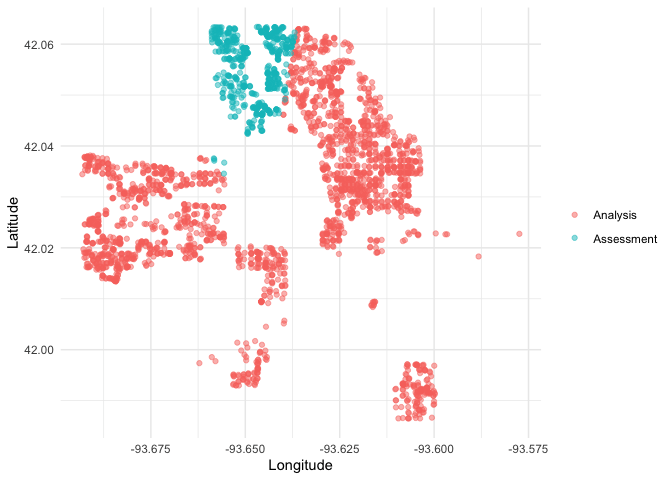## Introduction

The goal of spatialsample is to provide functions and classes for spatial resampling to use with rsample, including:

The implementation of more spatial resampling approaches is planned.

Like rsample, spatialsample provides building blocks for creating and analyzing resamples of a spatial data set but does not include code for modeling or computing statistics. The resampled data sets created by spatialsample are efficient and do not have much memory overhead.

## Installation

You can install the released version of spatialsample from CRAN with:

install.packages("spatialsample")

And the development version from GitHub with:

# install.packages("devtools")
devtools::install_github("tidymodels/spatialsample")

## Example

The most straightforward spatial resampling strategy with the lightest dependencies is spatial_clustering_cv(), which uses k-means clustering to identify cross-validation folds:

library(spatialsample)
data("ames", package = "modeldata")

set.seed(1234)
folds <- spatial_clustering_cv(ames, coords = c("Latitude", "Longitude"), v = 5)

folds
#> #  5-fold spatial cross-validation
#> # A tibble: 5 x 2
#>   splits             id
#>   <list>             <chr>
#> 1 <split [2332/598]> Fold1
#> 2 <split [2187/743]> Fold2
#> 3 <split [2570/360]> Fold3
#> 4 <split [2118/812]> Fold4
#> 5 <split [2513/417]> Fold5

In this example, the ames data on houses in Ames, IA is resampled with v = 5; notice that the resulting partitions do not contain an equal number of observations.

We can create a helper plotting function to visualize the five folds.

library(ggplot2)
library(purrr)
library(dplyr)
#>
#> Attaching package: 'dplyr'
#> The following objects are masked from 'package:stats':
#>
#>     filter, lag
#> The following objects are masked from 'package:base':
#>
#>     intersect, setdiff, setequal, union

plot_splits <- function(split) {
p <- analysis(split) %>%
mutate(analysis = "Analysis") %>%
bind_rows(assessment(split) %>%
mutate(analysis = "Assessment")) %>%
ggplot(aes(Longitude, Latitude, color = analysis)) +
geom_point(alpha = 0.5) +
labs(color = NULL)
print(p)
}

walk(folds\$splits, plot_splits)## Contributing

This project is released with a Contributor Code of Conduct. By contributing to this project, you agree to abide by its terms.